# GLM國產大模型訓練加速：效能最高提升3倍，視訊記憶體節省1/3，低成本上手2017 年，Google 提出了 Transformer 架構，隨後 BERT 、GPT、T5等預訓練模型不斷湧現，並在各項任務中都不斷重新整理 SOTA 紀錄。去年，清華提出了 GLM 模型（ https://github.com/THUDM/GLM ），不同於上述預訓練模型架構，它採用了一種自迴歸的空白填充方法， 在 NLP 領域三種主要的任務（自然語言理解、無條件生成、有條件生成）上都取得了不錯的結果。

• One-GLM： https://github.com/Oneflow-Inc/one-glm

• OneFlow： https://github.com/Oneflow-Inc/oneflow

## 1GLM-large 訓練效能和視訊記憶體的表現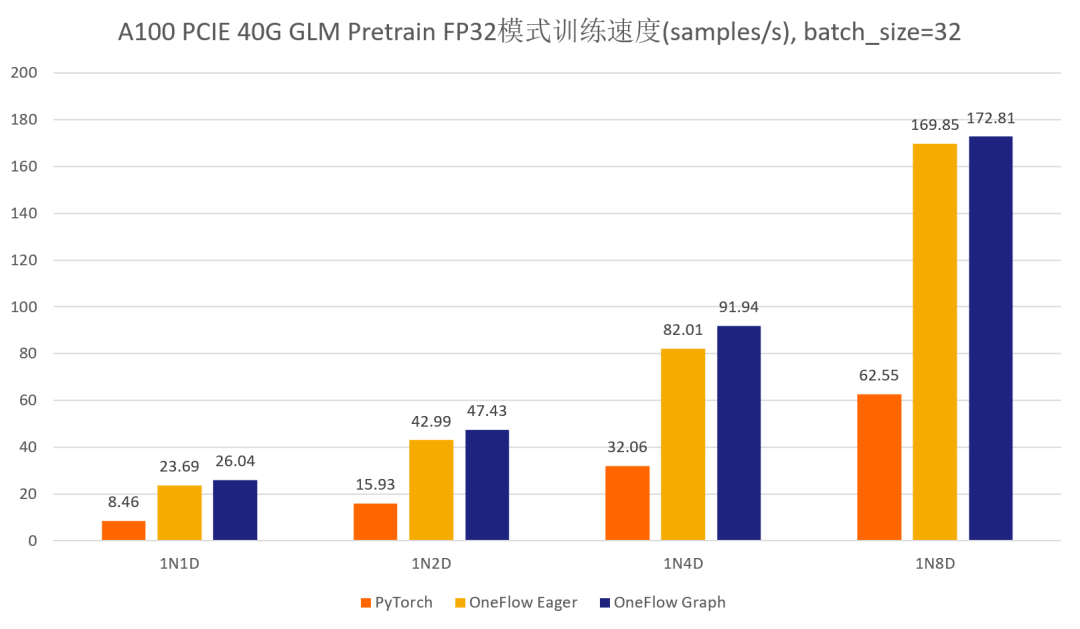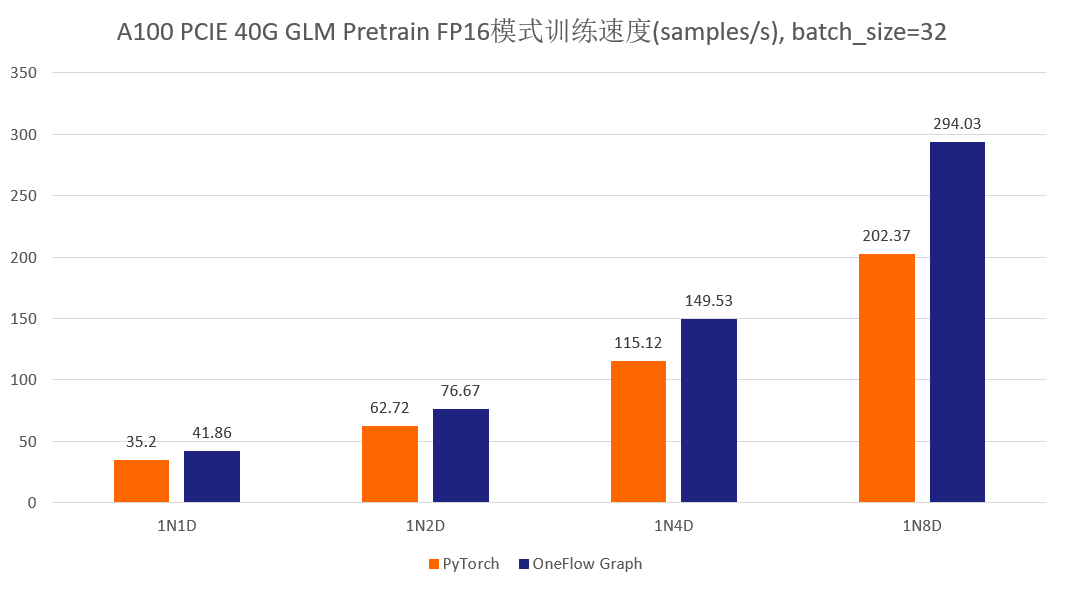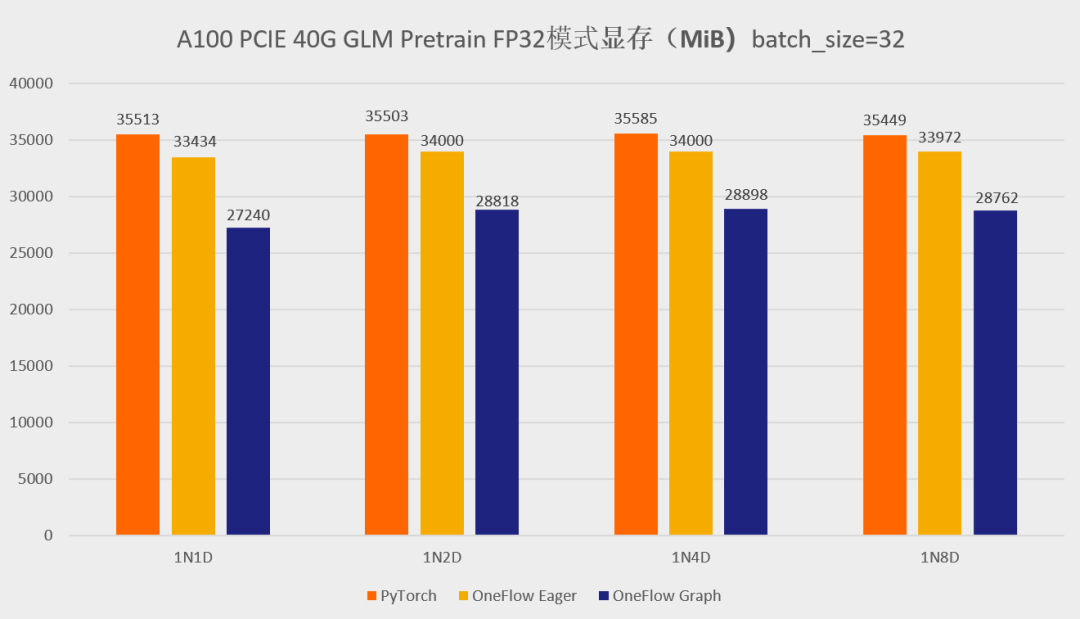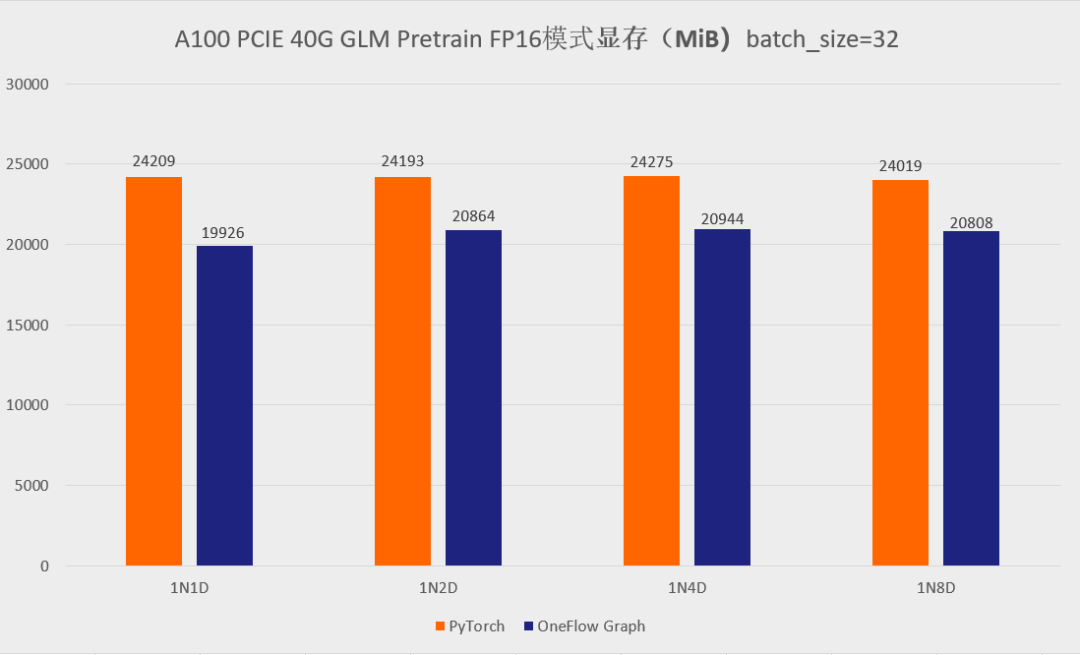## GLM 遷移，只需修改幾行程式碼

• 將 import torch 替換為  import oneflow as torch

• 將 import torch.xx 替換為 import oneflow.xx

• 將 from apex.normalization.fused_layer_norm import FusedLayerNorm as LayerNorm 替換為 from oneflow.nn import LayerNorm

• 註釋掉 torch.distributed.ReduceOp，torch.distributed.new_group,，torch.distributed.TCPStore，torch.distributed.all_reduce 這些API，它們是 PyTorch DDP 所需要的，但 OneFlow 的資料並行是由內部的 SBP 和 Global Tensor 機制實現，並不需要這些 API。

## 兩大調優手段

### loss 計算部分的優化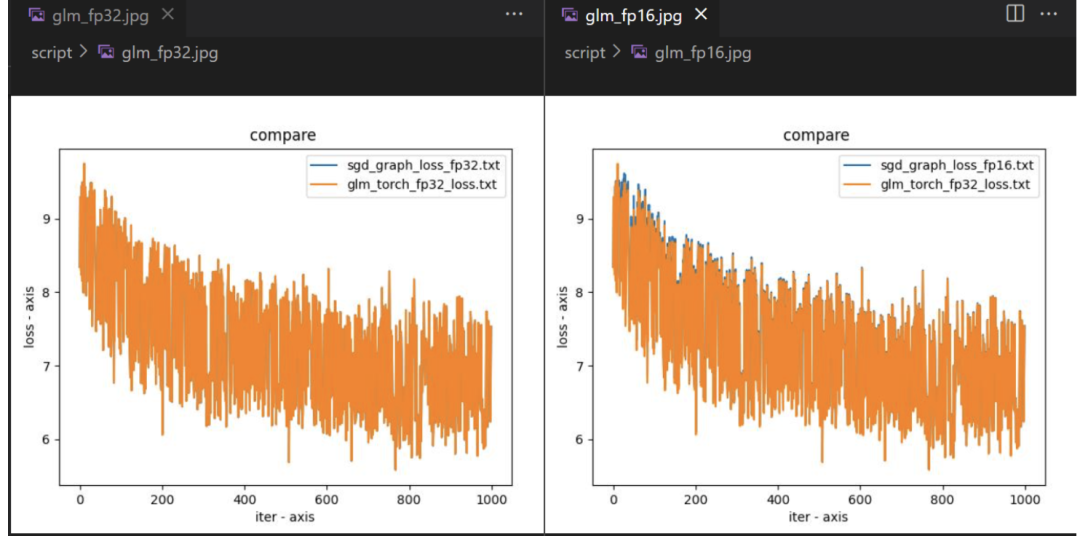## 4LiBai 也能輕鬆搞定 GLM 推理

### 分散式推理具有天然優勢

` `

`class Linear1D(nn.Module):` ` def __init__(self, in_features, out_features, parallel="data", layer_idx=0, ...):` ` super().__init__()`
`if parallel == "col":` ` weight_sbp = dist.get_nd_sbp([flow.sbp.broadcast, flow.sbp.split(0)])` ` elif parallel == "row":` ` weight_sbp = dist.get_nd_sbp([flow.sbp.broadcast, flow.sbp.split(1)])` ` elif parallel == "data":` ` weight_sbp = dist.get_nd_sbp([flow.sbp.broadcast, flow.sbp.broadcast])` ` else:` ` raise KeyError(f"{parallel} is not supported! Only support ('data', 'row' and 'col')")`
`self.weight = flow.nn.Parameter(` ` flow.empty(` ` (out_features, in_features),` ` dtype=flow.float32,` ` placement=dist.get_layer_placement(layer_idx), # for pipeline parallelism placement` ` sbp=weight_sbp,` ` )` ` )` ` init_method(self.weight)` ` ...` ` ` ` def forward(self, x):` ` ...`

### GLM 推理的 Demo 演示

• 單卡 generate 任務，我們選擇 glm-10b 模型：

` `
` `

`python demo.py`

` `

`# demo.py` `import oneflow as flow` `from projects.GLM.tokenizer.glm_tokenizer import GLMGPT2Tokenizer` `from libai.utils import distributed as dist` `from projects.GLM.configs.glm_inference import cfg` `from projects.GLM.modeling_glm import GLMForConditionalGeneration` `from projects.GLM.utils.glm_loader import GLMLoaderHuggerFace` `from omegaconf import DictConfig`
`tokenizer = GLMGPT2Tokenizer.from_pretrained("/data/home/glm-10b")` `input_ids = tokenizer.encode(` ` [` ` "Ng is an adjunct professor at [MASK] (formerly associate professor and Director of its Stanford AI Lab or SAIL ). Also a pioneer in online education, Ng co-founded Coursera and deeplearning.ai."` ` ],` ` return_tensors="of",` `)` `inputs = {"input_ids": input_ids, "attention_mask": flow.ones(input_ids.size())}` `inputs = tokenizer.build_inputs_for_generation(inputs, max_gen_length=512)`
`sbp = dist.get_nd_sbp([flow.sbp.broadcast, flow.sbp.broadcast])` `placement = dist.get_layer_placement(0)`
`dist.set_device_type("cpu")` `loader = GLMLoaderHuggerFace(GLMForConditionalGeneration, cfg, "/path/to/glm-10b")` `model = loader.load()` `model = model.half().cuda()`
`dist.set_device_type("cuda")` `outputs = model.generate(` ` inputs=inputs['input_ids'].to_global(sbp=sbp, placement=placement), ` ` position_ids=inputs['position_ids'].to_global(sbp=sbp, placement=placement), ` ` generation_attention_mask=inputs['generation_attention_mask'].to_global(sbp=sbp, placement=placement).half(), ` ` max_length=512` `)` `res = tokenizer.decode(outputs)` `print(res)` `>>> [CLS] Ng is an adjunct professor at [MASK] (formerly associate professor and Director of its Stanford AI Lab or SAIL ). Also a pioneer in online education, Ng co-founded Coursera and deeplearning.ai.<|endoftext|> <|startofpiece|> Stanford University and a co-founder of <|endofpiece|>`

``````
``````

• 4卡 model parallel+pipeline parallel generate 任務，選擇 glm-10b 模型：

` `
` `

`python3 -m oneflow.distributed.launch --nproc_per_node 4 demo.py`

` `

`# demo.py` `import oneflow as flow` `from projects.GLM.tokenizer.glm_tokenizer import GLMGPT2Tokenizer` `from libai.utils import distributed as dist` `from projects.GLM.configs.glm_inference import cfg` `from projects.GLM.modeling_glm import GLMForConditionalGeneration` `from projects.GLM.utils.glm_loader import GLMLoaderHuggerFace` `from omegaconf import DictConfig`
`# 只需簡單配置並行方案` `parallel_config = DictConfig(` ` dict(` ` data_parallel_size=1,` ` tensor_parallel_size=2,` ` pipeline_parallel_size=2,` ` pipeline_num_layers=2 * 24` ` )` `)` `dist.setup_dist_util(parallel_config)`
`tokenizer = GLMGPT2Tokenizer.from_pretrained("/data/home/glm-10b")` `input_ids = tokenizer.encode(` ` [` ` "Ng is an adjunct professor at [MASK] (formerly associate professor and Director of its Stanford AI Lab or SAIL ). Also a pioneer in online education, Ng co-founded Coursera and deeplearning.ai."` ` ],` ` return_tensors="of",` `)` `inputs = {"input_ids": input_ids, "attention_mask": flow.ones(input_ids.size())}` `inputs = tokenizer.build_inputs_for_generation(inputs, max_gen_length=512)`
`sbp = dist.get_nd_sbp([flow.sbp.broadcast, flow.sbp.broadcast])` `placement = dist.get_layer_placement(0)`
`loader = GLMLoaderHuggerFace(GLMForConditionalGeneration, cfg, "/path/to/glm-10b")` `model = loader.load()`
`outputs = model.generate(` ` inputs=inputs['input_ids'].to_global(sbp=sbp, placement=placement), ` ` position_ids=inputs['position_ids'].to_global(sbp=sbp, placement=placement), ` ` generation_attention_mask=inputs['generation_attention_mask'].to_global(sbp=sbp, placement=placement), ` ` max_length=512` `)` `res = tokenizer.decode(outputs)` `if dist.is_main_process():` ` print(res)` `>>> [CLS] Ng is an adjunct professor at [MASK] (formerly associate professor and Director of its Stanford AI Lab or SAIL ). Also a pioneer in online education, Ng co-founded Coursera and deeplearning.ai.<|endoftext|> <|startofpiece|> Stanford University and a co-founder of <|endofpiece|>`

• 使用 One- GLM 訓練的模型進行推理

## 結語

• One-GLM： https://github.com/Oneflow-Inc/one-glm

• OneFlow： https://github.com/Oneflow-Inc/oneflow

## 歡迎Star、試用OneFlow最新版本：https://github.com/Oneflow-Inc/oneflow/